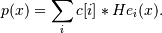# numpy.polynomial.hermite_e.hermeroots¶

numpy.polynomial.hermite_e.hermeroots(c)[source]

Compute the roots of a HermiteE series.

Return the roots (a.k.a. “zeros”) of the polynomialParameters: c : 1-D array_like 1-D array of coefficients. out : ndarray Array of the roots of the series. If all the roots are real, then out is also real, otherwise it is complex.

polyroots, legroots, lagroots, hermroots, chebroots

Notes

The root estimates are obtained as the eigenvalues of the companion matrix, Roots far from the origin of the complex plane may have large errors due to the numerical instability of the series for such values. Roots with multiplicity greater than 1 will also show larger errors as the value of the series near such points is relatively insensitive to errors in the roots. Isolated roots near the origin can be improved by a few iterations of Newton’s method.

The HermiteE series basis polynomials aren’t powers of x so the results of this function may seem unintuitive.

Examples

>>> from numpy.polynomial.hermite_e import hermeroots, hermefromroots
>>> coef = hermefromroots([-1, 0, 1])
>>> coef
array([ 0.,  2.,  0.,  1.])
>>> hermeroots(coef)
array([-1.,  0.,  1.])


#### Previous topic

numpy.polynomial.hermite_e.hermegrid3d

#### Next topic

numpy.polynomial.hermite_e.hermefromroots